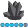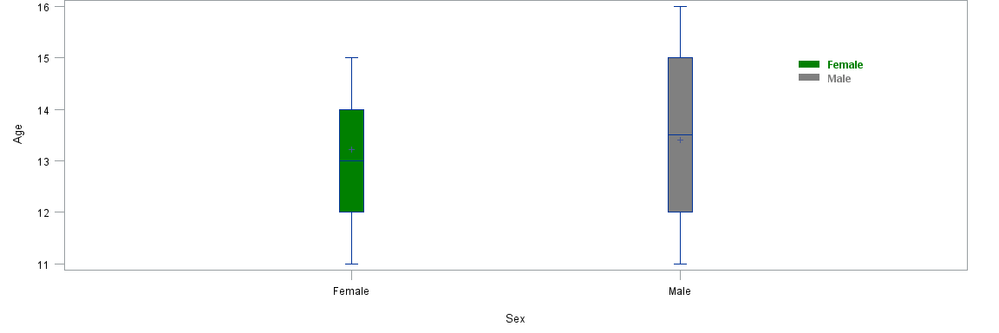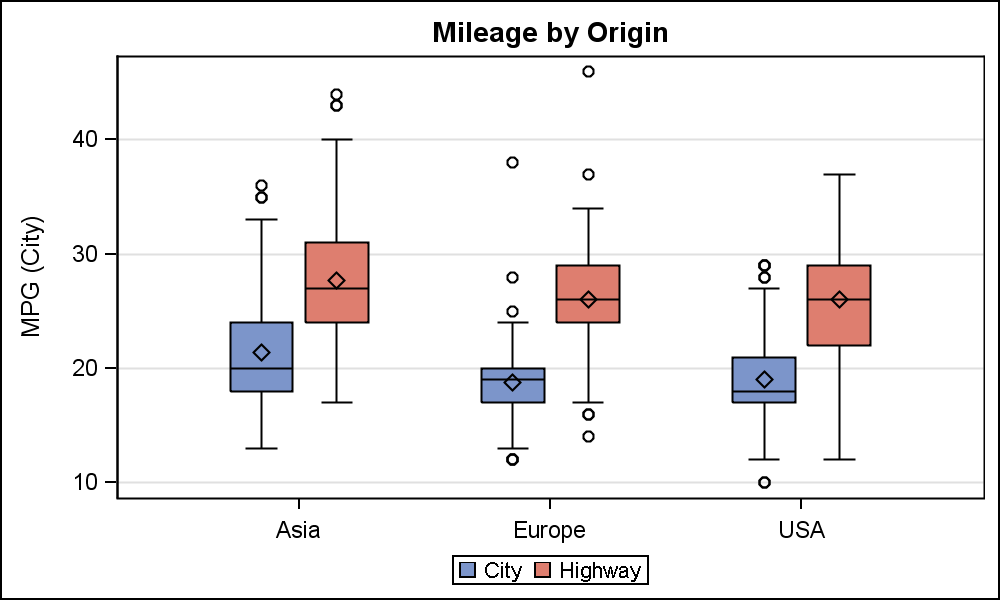## proc boxplot legend

Hi,

I am creating a boxplot comparing 2 Trt arms, side by side, on different visits.

I am differentiating two arms by different colors. Now I want to create a legend to specify which color is for which arm. How can I do that?

I am not using overlay statement in proc boxplot.

I have four visits for TRT 1 . And same four visits for TRT 2. Visits are on xaxis I am creating 8 different values for visits in two TRT arms, 4 for each.

And then plotting these 8 values on xaxis , and then differing the TRT arms using cboxfill.

I have attached the graph for your reference.

Any help would be really appreciated.

Thanks

1 ACCEPTED SOLUTION

Accepted SolutionsAncaTilea
Pyrite | Level 9

## Re: proc boxplot legend

Hi.

If you don't use SAS 9.2 or higher, most likely you'll have to use the annotate to add the legend.

Here is an example - using the sashelp.class;

*create the group color;

data temp;

set sashelp.class;

if sex = "F" then color="green";

if sex = "M" then color = "gray";

run;

goptions reset = all;

; Create the Annotate data set ;

data anno;

length function color \$ 8 text \$ 25 style \$ 25;

xsys='3'; ysys='3';

/* Draw the first square */

color='green';

function='move'; x=80; y=82; output;

function='bar';  x=82; y=80; style='solid'; output;

/* Label the first square */

function='label'; x=83; y=81; position='6';

style="'Albany AMT/bold'"; size=1; text='Female'; output;

/* Draw the second square */

color='gray'; style='solid';

function='move'; x=80; y=78; output;

function='bar';  x=82; y=76; style='solid'; output;

/* Label the first square */

function='label'; x=83; y=77; position='6';

style="'Albany AMT/bold'"; ; size=1; text='Male'; output;

run;

proc sort data = temp;by sex;run;

proc format;

value  \$ gender "M" = "Male" "F" = "Female";quit;

goptions reset = all;

proc boxplot data = temp anno = anno;

plot age*sex/boxstyle = schematic cboxfill = (color);

format sex \$gender.;

run;quit;

Best of luck!

Anca.5 REPLIES 5Jay54
Meteorite | Level 14

## Re: proc boxplot legend

Can you attach the full program including data?  Which release of SAS are you using?AncaTilea
Pyrite | Level 9

## Re: proc boxplot legend

Hi.

If you don't use SAS 9.2 or higher, most likely you'll have to use the annotate to add the legend.

Here is an example - using the sashelp.class;

*create the group color;

data temp;

set sashelp.class;

if sex = "F" then color="green";

if sex = "M" then color = "gray";

run;

goptions reset = all;

; Create the Annotate data set ;

data anno;

length function color \$ 8 text \$ 25 style \$ 25;

xsys='3'; ysys='3';

/* Draw the first square */

color='green';

function='move'; x=80; y=82; output;

function='bar';  x=82; y=80; style='solid'; output;

/* Label the first square */

function='label'; x=83; y=81; position='6';

style="'Albany AMT/bold'"; size=1; text='Female'; output;

/* Draw the second square */

color='gray'; style='solid';

function='move'; x=80; y=78; output;

function='bar';  x=82; y=76; style='solid'; output;

/* Label the first square */

function='label'; x=83; y=77; position='6';

style="'Albany AMT/bold'"; ; size=1; text='Male'; output;

run;

proc sort data = temp;by sex;run;

proc format;

value  \$ gender "M" = "Male" "F" = "Female";quit;

goptions reset = all;

proc boxplot data = temp anno = anno;

plot age*sex/boxstyle = schematic cboxfill = (color);

format sex \$gender.;

run;quit;

Best of luck!

Anca.## Re: proc boxplot legend

Thanks . Finally I am using annotation. I wish SAS would have some defined functionality to do this. BTW I am using SAS 9.2Jay54
Meteorite | Level 14

## Re: proc boxplot legend

With SAS 9.2M3, you can do this using GTL.proc template;
define statgraph cars;
begingraph;
entrytitle 'Mileage by Origin';
layout overlay / xaxisopts=(display=(ticks tickvalues))

yaxisopts=(griddisplay=on) cycleattrs=true;
boxplot x=origin y=mpg_city / discreteoffset=-0.15    boxwidth=0.25

name='c' legendlabel='City';
boxplot x=origin y=mpg_highway / discreteoffset= 0.15 boxwidth=0.25

name='h' legendlabel='Highway';
discretelegend 'c' 'h';
endlayout;
endgraph;
end;
run;

proc sgrender data=sashelp.cars(where=(type ne 'Hybrid')) template=cars;
run;

## Re: proc boxplot legend

Thanks Sanjay. Actually I was creating multiple graphs n same page. So I am not sure if I could have used it. For now, I have used annotation. Will explore this too for sure.

Thanks!

Discussion stats
• 5 replies
• 5486 views
• 1 like
• 3 in conversation